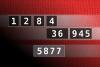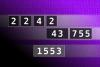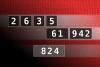BRAIN TEASERSBrain Teasers User Profile

# Alexander Filimonoff

rank
33
points
1564
See full ranking list
short ranking list
 31 Fuad Khalil Ibraheem 1739 32 Thea Ferlazzo 1724 33 Alexander Filimonoff 1564 34 FC Viñas 1479 35 Rajendra Talkute 1440
 Calculate the number 5877 NUMBERMANIA: Calculate the number 5877 using numbers [1, 2, 8, 4, 36, 945] and basic arithmetic operations (+, -, *, /). Each of the numbers can be used only once.Calculate the number 1553 NUMBERMANIA: Calculate the number 1553 using numbers [2, 2, 4, 2, 43, 755] and basic arithmetic operations (+, -, *, /). Each of the numbers can be used only once.Calculate the number 824 NUMBERMANIA: Calculate the number 824 using numbers [2, 6, 3, 5, 61, 942] and basic arithmetic operations (+, -, *, /). Each of the numbers can be used only once.